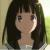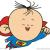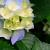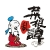## 关于jfinal类型转换的问题

@JFinal 你好，想跟你请教个问题：在使用jfinal的过程发现了几个使用不顺的地方，可能是自己使用过程中有点问题吧。

int id = r.getInt("xxid");获得.结果都是报错，说是字符串不能转换成int类型，还必须用

int id = Integer.parseInt(r.getInt("xxid").toString);来转换。还有类似的情况。通过页面上获取都是字符串形式的吧。看到源码中时这样定义的：

(Integer)columns.get(column); 直接用Integer转是否有些牵强呢？ 新手，望指导。谢谢

2```/**
* 类型柔化
* 主要用于基本类型间的转换操作,具有操作方便、容错性高、侵入性小的优点。
*/
public class ZObject
implements Serializable {

private static final long serialVersionUID = 1L;
/**
* 源对象
*/
private Object obj = null;

/**
* 类型柔化，源对象为 null
*/
public ZObject() {
}

/**
* 类型柔化
* @param obj 源对象
*/
public ZObject(Object obj) {
this.obj = obj;
}

/**
* 强制转换源对象类型
* 本方法提供仅为统一格式，并无特别之处
* @param <T>  对象类型
* @return
*/
@SuppressWarnings("unchecked")
public <T> T toObject() {
return (T) this.obj;
}

/**
* 获取源对象
* @return
*/
public Object getObject() {
return this.obj;
}

/**
* 静态转换
* @param obj  源对象
* @return
*/
public static ZObject conv(Object obj) {
return new ZObject(obj);
}
/**
* 布尔型
*/
public static final boolean TO_TYPE_BOOLEAN = Boolean.FALSE;
/**
* 字符串
*/
public static final Class<String> TO_TYPE_STRING = String.class;
/**
* 短整数
*/
public static final Class<Short> TO_TYPE_SHORT = Short.class;
/**
* 整型
*/
public static final Class<Integer> TO_TYPE_INT = Integer.class;
/**
* 长整
*/
public static final Class<Long> TO_TYPE_LONG = Long.class;
/**
* 浮点
*/
public static final Class<Float> TO_TYPE_FLOAT = Float.class;
/**
* 双精度
*/
public static final Class<Double> TO_TYPE_DOUBLE = Double.class;

/**
* 转换为其他类型。<br />
* 注意：如目标类型不可在以下类型中则进行强制转换，强制转换失败返回{@code null}
* @see #TO_TYPE_BOOLEAN
* @see #TO_TYPE_STRING
* @see #TO_TYPE_SHORT
* @see #TO_TYPE_INT
* @see #TO_TYPE_LONG
* @see #TO_TYPE_FLOAT
* @see #TO_TYPE_DOUBLE
* @param <T> 目标类型
* @param clazz 目标类型
* @return
*/
@SuppressWarnings("unchecked")
public <T> T toType(Class<T> clazz) {
/**
*
*/
if (String.class.isAssignableFrom(clazz)) {
return (T) toString();
}
/**
*
*/
if (Boolean.class.isAssignableFrom(clazz) || boolean.class.isAssignableFrom(clazz)) {
return (T) toBoolean();
}
/**
*
*/
if (Short.class.isAssignableFrom(clazz) || short.class.isAssignableFrom(clazz)) {
return (T) toShort();
}
/**
*
*/
if (Integer.class.isAssignableFrom(clazz) || int.class.isAssignableFrom(clazz)) {
return (T) toInteger();
}

/**
*
*/
if (Long.class.isAssignableFrom(clazz) || long.class.isAssignableFrom(clazz)) {
return (T) toLong();
}
/**
*
*/
if (Float.class.isAssignableFrom(clazz) || float.class.isAssignableFrom(clazz)) {
return (T) toFloat();
}
/**
*
*/
if (Double.class.isAssignableFrom(clazz) || double.class.isAssignableFrom(clazz)) {
return (T) toDouble();
}
/**
* 强制转换，失败则返回null
*/
try {
return clazz == null ? null : clazz.cast(obj);
} catch (ClassCastException ex) {
return null;
}
}

/**
* 转换为字符串
* @return  源对象为null时返回<b>空字符串("")</b>，而<b>不是</b>null
*/
@Override
public String toString() {
String _str = null;
do {
if (obj == null) {
break;
}
if (obj instanceof String) {
_str = obj.toString();
break;
}
_str = obj.toString();
} while (false);
return _str == null ? "" : _str;
}

/**
* 转换为字符
* @return  默认返回 0
*/
public char toChar() {
char _char = Character.UNASSIGNED;
String _str = this.toString();
if (_str != null && _str.length() > 0) {
_char = _str.charAt(0);
}
return _char;
}

/**
* 转换为字节
* @return 默认返回 0
*/
public byte toByte() {
byte _byte = 0;
String _str = this.toString();

if (_str != null && _str.getBytes().length > 0) {
_byte = _str.getBytes();
}
return _byte;
}

/**
* 转换为短整型
* @return 默认返回 0
*/
public Short toShort() {
short _short = 0;
int _int = toInteger();
if (_int <= Short.MAX_VALUE && _int >= Short.MIN_VALUE) {
_short = (short) _int;
}
return _short;
}

/**
* 验证十六进制字母
* @param ch
* @return
*/
private boolean isHex(char ch) {
if ((ch >= 'a' && ch <= 'f') || ch >= 'A' && ch <= 'F') {
return true;
}
return false;
}
private static final int NUM_TYPE_INT = 0; //
private static final int NUM_TYPE_LONG = 1; //
private static final int NUM_TYPE_FLOAT = 2; //
private static final int NUM_TYPE_DOUBLE = 3; //

/**
* 分析数字
* @param numStr    数字字符串
* @param isDot     是否有小数点
* @param isE       是否使用科学计数法
* @return
*/
private Number parseNum(int numtype) {
if (obj == null) {
return 0;
}
if (obj instanceof Boolean) {
return (Boolean.TRUE.equals(obj)) ? 1 : 0;
}
String numStr = StringHelper.ltrim(toString());
int len = numStr.length();
if (len == 0) {
return 0;
}
boolean isDot = (numtype == NUM_TYPE_FLOAT || numtype == NUM_TYPE_DOUBLE);
boolean isE = numtype == NUM_TYPE_DOUBLE;
int eIndex = -1; // e 位置（是否使用科学计数法）
int start = -1; // 数字起始位置（用于判别小数点位置是否合法）
int dotPos = -1; // 小数点位置
char ch = 0;
char tmpCh = 0;
StringBuilder numBuilder = new StringBuilder();
boolean isHex = false;
int i = 0;
/**
* int,long,float,double    hex
* float,double dot
* double   scientific notation
*/
if (numStr.charAt(0) == '+') {
i++;
}
if (numStr.charAt(0) == '-') {
i++;
numBuilder.append('-');
}
if (len >= i + 2) {
tmpCh = numStr.charAt(i + 1);
if (numStr.charAt(i) == '0'//
&& (tmpCh == 'x' || tmpCh == 'X')) {
isHex = true;
i += 2;
}
}
start = i;

for (; i < len; i++) {
ch = numStr.charAt(i);
if (isE) {
if (i != 0 && (ch == 'e' || ch == 'E') && !isHex && eIndex < 0) {
numBuilder.append(ch);
eIndex = i;
continue;
}
if (eIndex == i - 1 && eIndex > -1) {
if (ch == '-' || ch == '+') {
numBuilder.append(ch);
continue;
}
}
}
if (Character.isDigit(ch)) {
numBuilder.append(ch);
continue;
}
if (isHex) {
if (isHex(ch)) {
numBuilder.append(ch);
continue;
}
} else if (isDot) {
if (ch == '.') {
// 1.2
if (dotPos < 0 && start != i) {
numBuilder.append(ch);
dotPos = i;
continue;
} else {    // .12 , 1.2.3
break;
}
}
}
break;
}
int numLen = numBuilder.length();
if (numLen == 0) {
return 0;
}
tmpCh = numBuilder.charAt(0);
if (numLen == 1
&& (tmpCh == '+' //
|| tmpCh == '-')) {
return 0;
}
Number num = null;
if (isHex) {
switch (numtype) {
case NUM_TYPE_INT:
num = Integer.parseInt(numBuilder.toString(), 16);
break;
case NUM_TYPE_LONG:
case NUM_TYPE_FLOAT:
case NUM_TYPE_DOUBLE:
num = Long.parseLong(numBuilder.toString(), 16);
break;
}
} else {
switch (numtype) {
case NUM_TYPE_INT:
num = Integer.parseInt(numBuilder.toString());
break;
case NUM_TYPE_LONG:
num = Long.parseLong(numBuilder.toString());
break;
case NUM_TYPE_FLOAT:
num = Float.parseFloat(numBuilder.toString());
break;
case NUM_TYPE_DOUBLE:
num = Double.parseDouble(numBuilder.toString());
break;
}
}
return num == null ? 0 : num;
}

/**
* 转换为整型
* @return 默认返回 0
*/
public Integer toInteger() {
int _int = 0;
try {
_int = parseNum(NUM_TYPE_INT).intValue();
} catch (NumberFormatException nfex) {
/**
* 超过上限改为 0
* _integer = Integer.MAX_VALUE;
*/
_int = 0;
}
return _int;
}

/**
* 转换为布尔型，非0数字，不为空的字符串返回true
* @return  默认返回 false
*/
public Boolean toBoolean() {
boolean _boolean = false;
do {
if (obj == null) {
break;
}
/**
* bug: boolean string will be trigger stack error!
* fixed!
*/
if (obj instanceof Boolean) {
_boolean = Boolean.TRUE.equals(obj);
break;
}
String boolString = new ZObject(obj).toString();
Integer boolInteger = new ZObject(obj).toInteger();
if (boolInteger != 0) {
_boolean = true;
break;
}
if ("0".equals(boolString)
|| "false".equalsIgnoreCase(boolString)) {
break;
}
if (boolString.length() > 0) {
_boolean = true;
break;
}
} while (false);
return _boolean;
}

/**
* 转换为整数后再转换为字符串
* @return 默认返回 "0"
*/
public String toIntString() {
return String.valueOf(toInteger());
}

/**
* 转换为长整型
* @return 默认返回 0
*/
public Long toLong() {
long _long = 0L;

try {
_long = parseNum(NUM_TYPE_LONG).longValue();
} catch (NumberFormatException nfex) {
/**
* 超过上限改为 0
*  _long = Long.MAX_VALUE;
*/
_long = 0L; // 超过上限改为 0L
}
return _long;
}

/**
* 转换为浮点型
* @return 默认返回 0F
*/
public Float toFloat() {
float _float = 0F;
try {
_float = parseNum(NUM_TYPE_FLOAT).floatValue();
if (Float.isInfinite(_float) || Float.isNaN(_float)) {
return 0F;
}
} catch (NumberFormatException nfex) {
/**
* 超过上限改为 0
* _double = Integer.MAX_VALUE;
*/
_float = 0F;
}
return _float;
}

/**
* 转换为双精度
* @return 默认返回 0D
*/
public Double toDouble() {
double _double = 0D;
try {
_double = parseNum(NUM_TYPE_DOUBLE).doubleValue();
if (Double.isInfinite(_double) || Double.isNaN(_double)) {
return 0D;
}
} catch (NumberFormatException nfex) {
/**
* 超过上限改为 0
* _double = Integer.MAX_VALUE;
*/
_double = 0D;
}
return _double;
}
}
```

0#### 引用来自“谭明智”的答案

r是什么？
r是一个record。
00#### 引用来自“RobertCool”的答案

0#### 引用来自“谭明智”的答案

r是什么？
r是一个record。

0#### 引用来自“谭明智”的答案

r是什么？
r是一个record。

0#### 引用来自“谭明智”的答案

r是什么？
r是一个record。